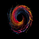# Volatility Switch Indicator [LazyBear]

צפיות ‎11459‎
The Volatility Switch (VOLSWITCH) indicator, by Ron McEwan, estimates current volatility in respect to historical data, thus indicating whether the market is trending or in mean reversion mode. Range is normalized to 0 - 1.

When Volatility Switch rises above the 0.5 level, volatility in the market is increasing, thus the price action can be expected to become choppier with abrupt moves. When the indicator falls below the 0.5 level from recent high readings, volatility decreases, which may be considered a sign of trend formation.

Trading strategy as suggested by Ron McEwan is:
- If VOLSWITCH is less than 0.5, volatility decreases, which may be considered a sign of trend formation
- If VOLSWITCH is greater than 0.5, market is in high volatility mode. Can be choppy. Use RSI to look for OB/OS levels.

I have implemented support for 2 lengths (14 and 21) Note that, Pine doesn't support loops. Once it is introduced, I will publish an updated version.

Building a strategy out of this is straightforward (refer to my strategy explanation above), I strongly encourage new Pinescript coders to try to a plotarrow() based overlay indicator to get more familiar with Pine.

---------
The Volatility (Regime) Switch Indicator : http://traders.com/Documentation/FEEDbk_...

Complete list of my indicators:
-------------------------------------
//
// @author LazyBear
// List of all my indicators:
//
study(title="Volatility Switch [LazyBear]", shorttitle="VOLSWITCH_LB")
dr= roc(close,1)/sma(close,2)
dummy=input(false, title="------- Choose lengths below ----"), il21=input(true, title="21"), il14=input(false, title="14")

vola21=stdev(dr, 21)
vswitch21=((vola21 <= vola21 ) + (vola21 <= vola21 ) +   (vola21 <= vola21 ) +
(vola21 <= vola21 ) +  (vola21 <= vola21 ) + (vola21 <= vola21 ) +
(vola21 <= vola21 ) +  (vola21 <= vola21 ) +  (vola21 <= vola21 ) +
(vola21 <= vola21 ) + (vola21 <= vola21 ) +  (vola21 <= vola21 ) +
(vola21 <= vola21 ) +  (vola21 <= vola21 ) +  (vola21 <= vola21 ) +
(vola21 <= vola21 ) +  (vola21 <= vola21 ) +  (vola21 <= vola21 ) +
(vola21 <= vola21 ) +  (vola21 <= vola21 ) + 1) / 21

vola14=stdev(dr, 14)
vswitch14=((vola14 <= vola14 ) + (vola14 <= vola14 ) +   (vola14 <= vola14 ) +
(vola14 <= vola14 ) +  (vola14 <= vola14 ) + (vola14 <= vola14 ) +
(vola14 <= vola14 ) +  (vola14 <= vola14 ) +  (vola14 <= vola14 ) +
(vola14 <= vola14 ) + (vola14 <= vola14 ) +  (vola14 <= vola14 ) +
(vola14 <= vola14 ) + 1) / 14

hline(0.5, title="Median")
plot(il21?vswitch21:na, color=red, linewidth=2, title="VOLSWITCH_21")
plot(il14?vswitch14:na, color=green, linewidth=2, title="VOLSWITCH_14")

List of my free indicators: http://bit.ly/1LQaPK8
List of my indicators at Appstore: http://blog.tradingview.com/?p=970

## תגובותExcellent description. I will be giving VOLSWITCH a try.

Can't wait for the loops!
השבAwesome! Thank you very much. It looks promising to filter very trending / non-trending areas in my first charts using it.
השבChartArt
YW. Looking fwd to ur charts with tuned params :)
השבVery thankful for the info/indicators you make available..
השב